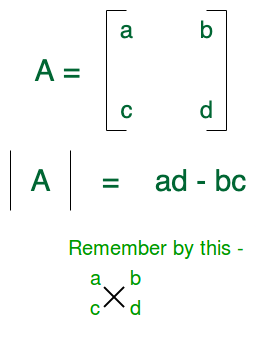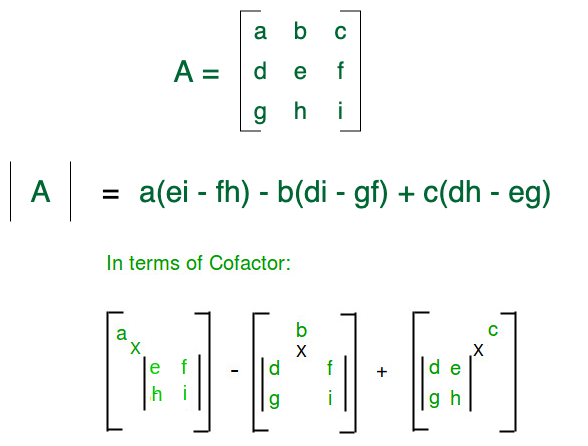Open in App
Not now

# Determinant of a Matrix

• Difficulty Level : Medium
• Last Updated : 18 Nov, 2022

The determinant of a Matrix is defined as a special number that is defined only for square matrices (matrices that have the same number of rows and columns). A determinant is used in many places in calculus and other matrices related to algebra, it actually represents the matrix in terms of a real number which can be used in solving a system of a linear equation and finding the inverse of a matrix.

Recommended Practice

## Determinant of 2 x 2 Matrix:Determinant of 2 x 2 matrix

## Determinant of 3 x 3 Matrix:Determinant of 3 x 3 matrix

## How to calculate?

The value of the determinant of a matrix can be calculated by the following procedure:

• For each element of the first row or first column get the cofactor of those elements.
• Then multiply the element with the determinant of the corresponding cofactor.
• Finally, add them with alternate signs. As a base case, the value of the determinant of a 1*1 matrix is the single value itself.

The cofactor of an element is a matrix that we can get by removing the row and column of that element from that matrix.

Code block

Output

`Determinant of the matrix is : 30`

Time Complexity: O(n4)
Space Complexity: O(n2), Auxiliary space used for storing cofactors.

Note: In the above recursive approach when the size of the matrix is large it consumes more stack size.

## Determinant of a Matrix using Determinant properties:

• In this method, we are using the properties of Determinant.
• Converting the given matrix into an upper triangular matrix using determinant properties
• The determinant of the upper triangular matrix is the product of all diagonal elements.
• Iterating every diagonal element and making all the elements down the diagonal as zero using determinant properties
• If the diagonal element is zero then search for the next non-zero element in the same column.

There exist two cases:

• Case 1: If there is no non-zero element. In this case, the determinant of a matrix is zero
• Case 2: If there exists a non-zero element there exist two cases
• Case A: If the index is with a respective diagonal row element. Using the determinant properties make all the column elements down to it zero
• Case B: Swap the row with respect to the diagonal element column and continue the Case A operation.

Below is the implementation of the above approach:

## C++

 `// C++ program to find Determinant of a matrix``#include ``using` `namespace` `std;` `// Dimension of input square matrix``#define N 4``// Function to get determinant of matrix``int` `determinantOfMatrix(``int` `mat[N][N], ``int` `n)``{``    ``int` `num1, num2, det = 1, index,``                    ``total = 1; ``// Initialize result` `    ``// temporary array for storing row``    ``int` `temp[n + 1];` `    ``// loop for traversing the diagonal elements``    ``for` `(``int` `i = 0; i < n; i++)``    ``{``        ``index = i; ``// initialize the index` `        ``// finding the index which has non zero value``        ``while` `(index < n && mat[index][i] == 0)``        ``{``            ``index++;``        ``}``        ``if` `(index == n) ``// if there is non zero element``        ``{``            ``// the determinant of matrix as zero``            ``continue``;``        ``}``        ``if` `(index != i)``        ``{``            ``// loop for swapping the diagonal element row and``            ``// index row``            ``for` `(``int` `j = 0; j < n; j++)``            ``{``                ``swap(mat[index][j], mat[i][j]);``            ``}``            ``// determinant sign changes when we shift rows``            ``// go through determinant properties``            ``det = det * ``pow``(-1, index - i);``        ``}` `        ``// storing the values of diagonal row elements``        ``for` `(``int` `j = 0; j < n; j++)``        ``{``            ``temp[j] = mat[i][j];``        ``}``        ``// traversing every row below the diagonal element``        ``for` `(``int` `j = i + 1; j < n; j++)``        ``{``            ``num1 = temp[i]; ``// value of diagonal element``            ``num2 = mat[j][i]; ``// value of next row element` `            ``// traversing every column of row``            ``// and multiplying to every row``            ``for` `(``int` `k = 0; k < n; k++)``            ``{``                ``// multiplying to make the diagonal``                ``// element and next row element equal``                ``mat[j][k]``                    ``= (num1 * mat[j][k]) - (num2 * temp[k]);``            ``}``            ``total = total * num1; ``// Det(kA)=kDet(A);``        ``}``    ``}` `    ``// multiplying the diagonal elements to get determinant``    ``for` `(``int` `i = 0; i < n; i++)``    ``{``        ``det = det * mat[i][i];``    ``}``    ``return` `(det / total); ``// Det(kA)/k=Det(A);``}` `// Driver code``int` `main()``{``    ``/*int mat[N][N] = {{6, 1, 1},``                        ``{4, -2, 5},``                        ``{2, 8, 7}}; */` `    ``int` `mat[N][N] = { { 1, 0, 2, -1 },``                      ``{ 3, 0, 0, 5 },``                      ``{ 2, 1, 4, -3 },``                      ``{ 1, 0, 5, 0 } };` `    ``// Function call``    ``printf``(``"Determinant of the matrix is : %d"``,``           ``determinantOfMatrix(mat, N));``    ``return` `0;``}`

## Java

 `// Java program to find Determinant of a matrix``class` `GFG``{` `    ``// Dimension of input square matrix``    ``static` `final` `int` `N = ``4``;` `    ``// Function to get determinant of matrix``    ``static` `int` `determinantOfMatrix(``int` `mat[][], ``int` `n)``    ``{``        ``int` `num1, num2, det = ``1``, index,``                        ``total = ``1``; ``// Initialize result` `        ``// temporary array for storing row``        ``int``[] temp = ``new` `int``[n + ``1``];` `        ``// loop for traversing the diagonal elements``        ``for` `(``int` `i = ``0``; i < n; i++)``        ``{``            ``index = i; ``// initialize the index` `            ``// finding the index which has non zero value``            ``while` `(index < n && mat[index][i] == ``0``)``            ``{``                ``index++;``            ``}``            ``if` `(index == n) ``// if there is non zero element``            ``{``                ``// the determinant of matrix as zero``                ``continue``;``            ``}``            ``if` `(index != i)``            ``{``                ``// loop for swapping the diagonal element row``                ``// and index row``                ``for` `(``int` `j = ``0``; j < n; j++)``                ``{``                    ``swap(mat, index, j, i, j);``                ``}``                ``// determinant sign changes when we shift``                ``// rows go through determinant properties``                ``det = (``int``)(det * Math.pow(-``1``, index - i));``            ``}` `            ``// storing the values of diagonal row elements``            ``for` `(``int` `j = ``0``; j < n; j++)``            ``{``                ``temp[j] = mat[i][j];``            ``}` `            ``// traversing every row below the diagonal``            ``// element``            ``for` `(``int` `j = i + ``1``; j < n; j++)``            ``{``                ``num1 = temp[i]; ``// value of diagonal element``                ``num2 = mat[j]``                          ``[i]; ``// value of next row element` `                ``// traversing every column of row``                ``// and multiplying to every row``                ``for` `(``int` `k = ``0``; k < n; k++)``                ``{``                    ``// multiplying to make the diagonal``                    ``// element and next row element equal``                    ``mat[j][k] = (num1 * mat[j][k])``                                ``- (num2 * temp[k]);``                ``}``                ``total = total * num1; ``// Det(kA)=kDet(A);``            ``}``        ``}` `        ``// multiplying the diagonal elements to get``        ``// determinant``        ``for` `(``int` `i = ``0``; i < n; i++)``        ``{``            ``det = det * mat[i][i];``        ``}``        ``return` `(det / total); ``// Det(kA)/k=Det(A);``    ``}` `    ``static` `int``[][] swap(``int``[][] arr, ``int` `i1, ``int` `j1, ``int` `i2,``                        ``int` `j2)``    ``{``        ``int` `temp = arr[i1][j1];``        ``arr[i1][j1] = arr[i2][j2];``        ``arr[i2][j2] = temp;``        ``return` `arr;``    ``}` `    ``// Driver code``    ``public` `static` `void` `main(String[] args)``    ``{``        ``/*int mat[N][N] = {{6, 1, 1},``                        ``{4, -2, 5},``                        ``{2, 8, 7}}; */` `        ``int` `mat[][] = { { ``1``, ``0``, ``2``, -``1` `},``                        ``{ ``3``, ``0``, ``0``, ``5` `},``                        ``{ ``2``, ``1``, ``4``, -``3` `},``                        ``{ ``1``, ``0``, ``5``, ``0` `} };` `        ``// Function call``        ``System.out.printf(``            ``"Determinant of the matrix is : %d"``,``            ``determinantOfMatrix(mat, N));``    ``}``}` `// This code is contributed by Rajput-Ji`

## Python3

 `# Python program to find Determinant of a matrix`  `def` `determinantOfMatrix(mat, n):` `    ``temp ``=` `[``0``]``*``n  ``# temporary array for storing row``    ``total ``=` `1``    ``det ``=` `1`  `# initialize result` `    ``# loop for traversing the diagonal elements``    ``for` `i ``in` `range``(``0``, n):``        ``index ``=` `i  ``# initialize the index` `        ``# finding the index which has non zero value``        ``while``(index < n ``and` `mat[index][i] ``=``=` `0``):``            ``index ``+``=` `1` `        ``if``(index ``=``=` `n):  ``# if there is non zero element``            ``# the determinant of matrix as zero``            ``continue` `        ``if``(index !``=` `i):``            ``# loop for swapping the diagonal element row and index row``            ``for` `j ``in` `range``(``0``, n):``                ``mat[index][j], mat[i][j] ``=` `mat[i][j], mat[index][j]` `            ``# determinant sign changes when we shift rows``            ``# go through determinant properties``            ``det ``=` `det``*``int``(``pow``(``-``1``, index``-``i))` `        ``# storing the values of diagonal row elements``        ``for` `j ``in` `range``(``0``, n):``            ``temp[j] ``=` `mat[i][j]` `        ``# traversing every row below the diagonal element``        ``for` `j ``in` `range``(i``+``1``, n):``            ``num1 ``=` `temp[i]     ``# value of diagonal element``            ``num2 ``=` `mat[j][i]   ``# value of next row element` `            ``# traversing every column of row``            ``# and multiplying to every row``            ``for` `k ``in` `range``(``0``, n):``                ``# multiplying to make the diagonal``                ``# element and next row element equal` `                ``mat[j][k] ``=` `(num1``*``mat[j][k]) ``-` `(num2``*``temp[k])` `            ``total ``=` `total ``*` `num1  ``# Det(kA)=kDet(A);` `    ``# multiplying the diagonal elements to get determinant``    ``for` `i ``in` `range``(``0``, n):``        ``det ``=` `det``*``mat[i][i]` `    ``return` `int``(det``/``total)  ``# Det(kA)/k=Det(A);`  `# Drivers code``if` `__name__ ``=``=` `"__main__"``:``    ``# mat=[[6 1 1][4 -2 5][2 8 7]]` `    ``mat ``=` `[[``1``, ``0``, ``2``, ``-``1``], [``3``, ``0``, ``0``, ``5``], [``2``, ``1``, ``4``, ``-``3``], [``1``, ``0``, ``5``, ``0``]]``    ``N ``=` `len``(mat)``    ` `    ``# Function call``    ``print``(``"Determinant of the matrix is : "``, determinantOfMatrix(mat, N))`

## C#

 `// C# program to find Determinant of a matrix``using` `System;` `class` `GFG {` `    ``// Dimension of input square matrix``    ``static` `readonly` `int` `N = 4;` `    ``// Function to get determinant of matrix``    ``static` `int` `determinantOfMatrix(``int``[, ] mat, ``int` `n)``    ``{``        ``int` `num1, num2, det = 1, index,``                        ``total = 1; ``// Initialize result` `        ``// temporary array for storing row``        ``int``[] temp = ``new` `int``[n + 1];` `        ``// loop for traversing the diagonal elements``        ``for` `(``int` `i = 0; i < n; i++)``        ``{``            ``index = i; ``// initialize the index` `            ``// finding the index which has non zero value``            ``while``(index < n && mat[index, i] == 0)``            ``{``                ``index++;``            ``}``            ``if` `(index == n) ``// if there is non zero element``            ``{``                ``// the determinant of matrix as zero``                ``continue``;``            ``}``            ``if` `(index != i)``            ``{``                ``// loop for swapping the diagonal element row``                ``// and index row``                ``for` `(``int` `j = 0; j < n; j++)``                ``{``                    ``swap(mat, index, j, i, j);``                ``}``                ``// determinant sign changes when we shift``                ``// rows go through determinant properties``                ``det = (``int``)(det * Math.Pow(-1, index - i));``            ``}` `            ``// storing the values of diagonal row elements``            ``for` `(``int` `j = 0; j < n; j++)``            ``{``                ``temp[j] = mat[i, j];``            ``}` `            ``// traversing every row below the diagonal``            ``// element``            ``for` `(``int` `j = i + 1; j < n; j++)``            ``{``                ``num1 = temp[i]; ``// value of diagonal element``                ``num2 = mat[j,``                           ``i]; ``// value of next row element` `                ``// traversing every column of row``                ``// and multiplying to every row``                ``for` `(``int` `k = 0; k < n; k++)``                ``{` `                    ``// multiplying to make the diagonal``                    ``// element and next row element equal``                    ``mat[j, k] = (num1 * mat[j, k])``                                ``- (num2 * temp[k]);``                ``}``                ``total = total * num1; ``// Det(kA)=kDet(A);``            ``}``        ``}` `        ``// multiplying the diagonal elements to get``        ``// determinant``        ``for` `(``int` `i = 0; i < n; i++)``        ``{``            ``det = det * mat[i, i];``        ``}``        ``return` `(det / total); ``// Det(kA)/k=Det(A);``    ``}` `    ``static` `int``[, ] swap(``int``[, ] arr, ``int` `i1, ``int` `j1, ``int` `i2,``                        ``int` `j2)``    ``{``        ``int` `temp = arr[i1, j1];``        ``arr[i1, j1] = arr[i2, j2];``        ``arr[i2, j2] = temp;``        ``return` `arr;``    ``}` `    ``// Driver code``    ``public` `static` `void` `Main(String[] args)``    ``{``        ``/*int mat[N,N] = {{6, 1, 1},``                        ``{4, -2, 5},``                        ``{2, 8, 7}}; */` `        ``int``[, ] mat = { { 1, 0, 2, -1 },``                        ``{ 3, 0, 0, 5 },``                        ``{ 2, 1, 4, -3 },``                        ``{ 1, 0, 5, 0 } };` `        ``// Function call``        ``Console.Write(``"Determinant of the matrix is : {0}"``,``                      ``determinantOfMatrix(mat, N));``    ``}``}` `// This code is contributed by 29AjayKumar`

## Javascript

 `Javascript`

Output

`Determinant of the matrix is : 30`

Time complexity: O(n3
Auxiliary Space: O(n), Space used for storing row.

## Determinant of a Matrix Using the NumPy package in Python

There is a built-in function or method in linalg module of NumPy package in python. It can be called numpy.linalg.det(mat) which returns the determinant value of the matrix mat passed in the argument.

## Python3

 `# importing the numpy package``# as np``import` `numpy as np` `def` `determinant(mat):``    ` `    ``# calling the det() method``    ``det ``=` `np.linalg.det(mat)``    ``return` `round``(det)` `# Driver Code``# declaring the matrix``mat ``=` `[[``1``, ``0``, ``2``, ``-``1``],``       ``[``3``, ``0``, ``0``, ``5``],``       ``[``2``, ``1``, ``4``, ``-``3``],``       ``[``1``, ``0``, ``5``, ``0``]]` `# Function call``print``(``'Determinant of the matrix is:'``,``      ``determinant(mat))`

Output:

`Determinant of the matrix is: 30`

Time Complexity: O(n3), as the time complexity of np.linalg.det is O(n3) for an n x n order matrix.
Auxiliary Space: O(1)

My Personal Notes arrow_drop_up